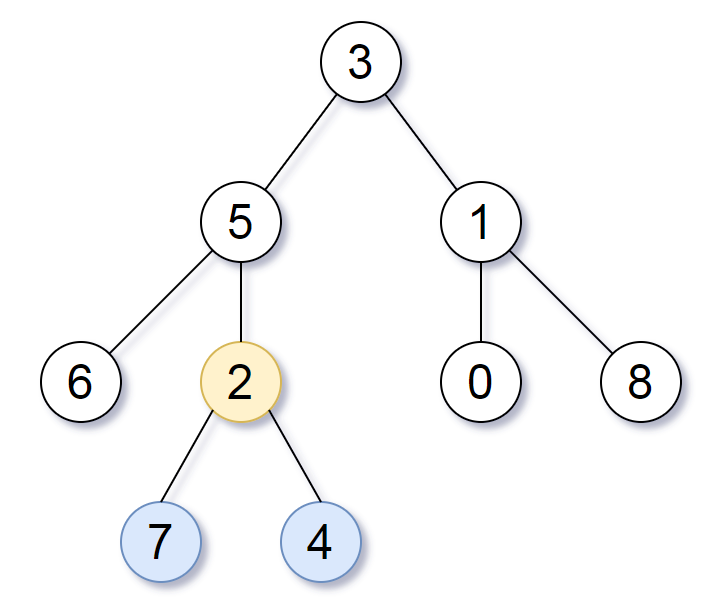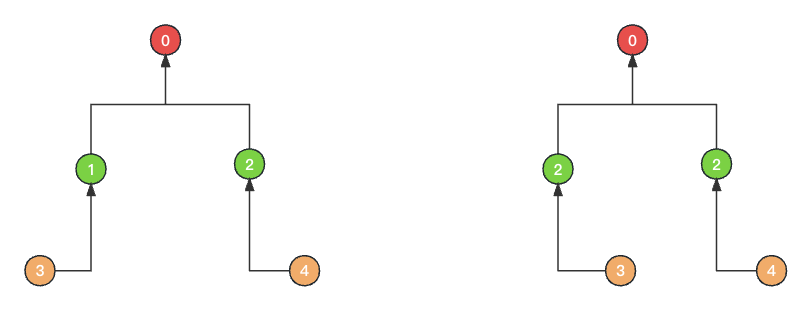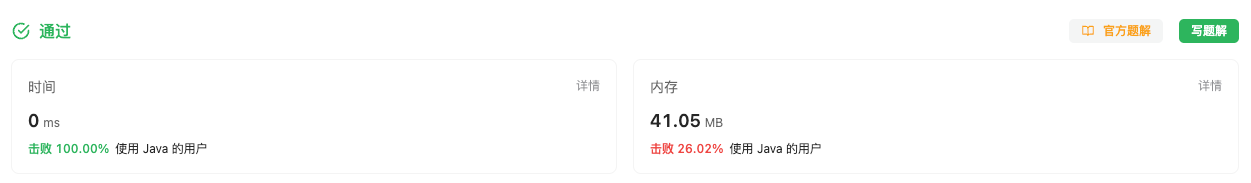#### 1123. 最深叶节点的最近公共祖先

###### 来源: 每日一题 2023.09.06

• 叶节点 是二叉树中没有子节点的节点

• 树的根节点的 深度`0`，如果某一节点的深度为 `d`，那它的子节点的深度就是 `d+1`

• 如果我们假定 `A` 是一组节点 `S`最近公共祖先`S` 中的每个节点都在以 `A` 为根节点的子树中，且 `A` 的深度达到此条件下可能的最大值。``````输入：root = [3,5,1,6,2,0,8,null,null,7,4]

``````

``````输入：root = 

``````

``````输入：root = [0,1,3,null,2]

``````

• 树中的节点数将在 `[1, 1000]` 的范围内。
• `0 <= Node.val <= 1000`
• 每个节点的值都是 独一无二 的。
``````/**
* Definition for a binary tree node.
* public class TreeNode {
*     int val;
*     TreeNode left;
*     TreeNode right;
*     TreeNode() {}
*     TreeNode(int val) { this.val = val; }
*     TreeNode(int val, TreeNode left, TreeNode right) {
*         this.val = val;
*         this.left = left;
*         this.right = right;
*     }
* }
*/
class Solution {

}
}
``````

#### 分析与题解

• 深度优先遍历

对于这个题目, 我们的想要的结果是一个二叉树的节点. 这个节点是最大深度的节点的公共最小祖先节点.

那么, 就会有如下的条件出现.

• 这个公共节点的 左子树右子树深度一定相等.

• 左子树的深度 、 右子树深度 、 最大深度三者一定相等.

只有如上的两个条件满足之后, 才能返回节点.

如下图所示. 不管 节点3 在左还是在右, 我们发现最小公共祖先节点依然是 节点0 ,并且我们可以通过图来验证上面的两个条件.然后, 我们利用深度优先遍历的思想, 遍历这个树并且维护整个树的最大深度. 如果上面的条件出现, 那么就是我们所需要的最小公共祖先节点了.

``````private int dfs(TreeNode root, int depth) {
if (root == null) {
maxDepth = Math.max(depth, maxDepth);
return depth;
}
int leftMaxDepth = dfs(root.left, depth+1);
int rightMaxDepth = dfs(root.right, depth+1);
if (leftMaxDepth == rightMaxDepth && leftMaxDepth == maxDepth) {
result = root;
}
return Math.max(leftMaxDepth, rightMaxDepth);
}
``````

整体的解题思路如下所示.

``````/**
* Definition for a binary tree node.
* public class TreeNode {
*     int val;
*     TreeNode left;
*     TreeNode right;
*     TreeNode() {}
*     TreeNode(int val) { this.val = val; }
*     TreeNode(int val, TreeNode left, TreeNode right) {
*         this.val = val;
*         this.left = left;
*         this.right = right;
*     }
* }
*/
class Solution {
TreeNode result;
int maxDepth = -1;
dfs(root, 0);
return result;
}
private int dfs(TreeNode root, int depth) {
if (root == null) {
maxDepth = Math.max(depth, maxDepth);
return depth;
}
int leftMaxDepth = dfs(root.left, depth+1);
int rightMaxDepth = dfs(root.right, depth+1);
if (leftMaxDepth == rightMaxDepth && leftMaxDepth == maxDepth) {
result = root;
}
return Math.max(leftMaxDepth, rightMaxDepth);
}
}
``````

复杂度分析:

• 时间复杂度: O(n), 时间复杂度与 二叉树的节点树成线性比例关系.
• 空间复杂度: O(n), `leftMaxDepth``rightMaxDepth` 的个数 与 二叉树的节点树成线性比例关系.

结果如下所示.IT界无底坑洞栋主 欢迎加Q骚扰:676758285